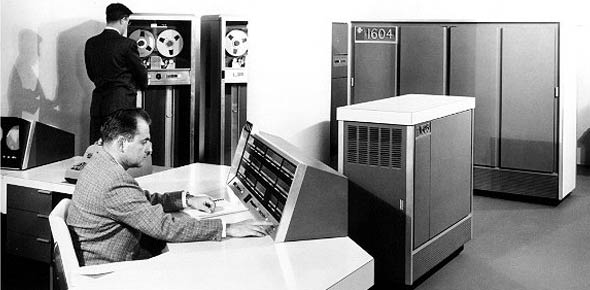# 2r051 CDC Volulme 3

20 Questions | Attempts: 185
ShareSettings5 Level CDC Volume 3

• 1.
1. What is the value of 5 / 2 x 6?
• A.

0.3

• B.

8.4

• C.

15.0

• D.

30.0

• 2.
2. Compute the difference of the signed numbers -17 -(-10).
• A.

+3.0

• B.

-3.0

• C.

-7.0

• D.

-27.0

• 3.
3. Eighty is 40 percent of what number?
• A.

200

• B.

320

• C.

400

• D.

500

• 4.
4. What statistical method uses only a sample of data?
• A.

Inferential

• B.

Descriptive

• C.

Data survey

• D.

Randomization

• 5.
5. Which action would require the use of the descriptive statistics method?
• A.

• B.

Choose a random sample of data.

• C.

Summarize a large amount of data.

• D.

Generalize from the results of small samples.

• 6.
6. In the equation Y=X+2, what does the symbol "Y" represent?
• A.

Exponent.

• B.

Inequality.

• C.

Subscript.

• D.

Variable.

• 7.
7. What is the value of 43?
• A.

64.

• B.

24.

• C.

12.

• D.

7.

• 8.
8. Which is the best example of a population in statistical language?
• A.

Yearly B-1 failures at Dyess AFB.

• B.

Weekly C-130 failures at Scott AFB.

• C.

Monthly E-3C failures at Tinker AFB.

• D.

Total T-38s failures across the Air Force

• 9.
9. A sample of a population that is taken in such a manner that each value has an equal chance of being selected is referred to as a
• A.

Biased sample.

• B.

Random sample.

• C.

Sampling theory.

• D.

Sampling application.

• 10.
10. What are measures, such as the mean or median, called if they are computed from a sample?
• A.

Statistics.

• B.

Parameter.

• C.

Population.

• D.

Distribution.

• 11.
11. Which measurement scale consist of equal intervals between scale values and an arbitrary zero point?
• A.

Ratio.

• B.

Nominal.

• C.

Ordinal.

• D.

Interval.

• 12.
12. You are given two pieces of test equipment that must be loaded on a pallet. Once piece weighs 125 pounds and the other piece weighs 3.5 times as much. Using the ratio measurement scale, how much does the second piece of equipment weight?
• A.

375.0 pounds.

• B.

415.5 pounds

• C.

437.5 pounds

• D.

500.0 pounds

• 13.
13. The second step in making a frequency distribution is to
• A.

Determine the range of the data.

• B.

Determine the class interval size.

• C.

Range the data from largest to smallest.

• D.

Rang the data from smallest to largest.

• 14.
14. Given the following noncumulative frequency distribution, which class interval will give you 18 classes?
• A.

0.1.

• B.

0.2.

• C.

0.3.

• D.

0.4.

• 15.
15. If you contruct a frequency distribution and the class interval is too large, the result is
• A.

A loss of detail.

• B.

Side gaps between items.

• C.

Overlapping lower limits.

• D.

A loss of smoothness and simplicity.

• 16.
16. When constructing a frequency polygon, what are plotted against the corresponding midpoints?
• A.

Series of rectangles.

• B.

Individual values of data.

• C.

Lower limit and baseline.

• D.

Frequencies of the various class intervals.

• 17.
17. Which graphical method gives the best representation of the number of individual values in each class?
• A.

Histogram.

• B.

Frequency polygon.

• C.

Overlapping polygon.

• D.

Overlapping histogram.

• 18.
18. What measure of central tendency is the most typical value in a distribution?
• A.

Mean.

• B.

Mode.

• C.

Median.

• D.

Weighted mean.

• 19.
19. What is the mode of a sample consisting of these values: 6,9,10,3,2,6 and 25?
• A.

2.

• B.

6.

• C.

8.

• D.

18.

• 20.
20. The median cannot be used with data from which measurement scale?
• A.

Nominal.

• B.

Interval.

• C.

Ordinal.

• D.

Ratio.

## Related TopicsBack to top
×

Wait!
Here's an interesting quiz for you.